Double-Slit Interference and Single-Slit Diffraction Experiments on Electrons

Double-Slit Interference and Single-Slit Diffraction Experiments on Electrons

Huawang Li

Abstract: De Broglie proposed the matter wave in 1924. The de Broglie wave is neither a mechanical wave nor an electromagnetic (EM) wave and has a very short wavelength. The Davisson-Germer electron diffraction experiment performed in 1925 involved bombarding the surface of a nickel crystal with a narrow beam of electrons. When the accelerating voltage V was maintained at 54 V, the wavelength of the incident electron was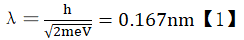,  demonstrating the existence of the matter wave. We introduce a type of electron wave with a very long wavelength in this study that is different from the matter wave. For example, the wavelength of the electron wave can reach 0.43 mm in the double-slit interference of electrons. Experiments demonstrate that this long-wavelength electron wave can produce both double-slit interference and electron diffraction. A comparative analysis of matter and electron waves reveals the physical natures of these waves and wave–particle duality.

Keywords: Double-slit electron interference, Electron diffraction, Wave-particle duality

Introduction

The discovery of long-wavelength electron interference and diffraction follows from multiple hypotheses. (1) The universe is filled with a gas of particles with an average kinetic energy equal to the Planck constant. This gas particle is referred to as a Yizi, , and the gas is called a Yizi gas. (2) Light is transmitted by the Yizi gas. As the average speed of a Yizi is approximately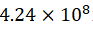m/s, the speed of light in a Yizi gas is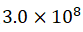m/s. (3) The moving mass of electrons and protons is equal to the static mass of these particles plus the mass of the Yizi gas displaced by these particles. The mass-energy equivalence is E = 1/2 × the mass of the Yizi gas displaced by electrons or protons × the square of the average speed of a Yizi. (4) Like protons, electrons and Yizi particles have point spherical surfaces that are both concave and convex. Particles participate in edge-edge collisions, that is, linear momentum and angular momentum are transferred at the same time. Protons, electrons, and Yizi particles undergo linear motion and eccentric rotation, and the motion trajectory of the center of mass (COM) in the respective rotational plane is a circular closed curve composed of periodic fluctuation curves, as shown in Figure 1. The linear motion of electrons from left to right is accompanied by eccentric rotation around Point P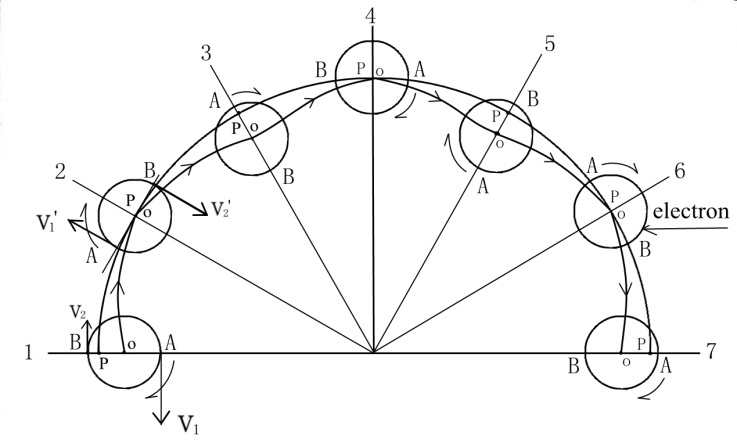Points A and B in the figure are two mass points with the same mass on the electron surface. At Position 1, the linear velocity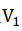of Point A in eccentric rotation around Point P is greater than the linear velocity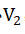of Point B rotating around Point P, such that Point A has a higher angular momentum than Point B. During the eccentric rotation of electrons, the angular momentum of Point A is gradually transferred to Point B, and vice versa. Point A, upon reaching Position 2, has the same angular momentum as Point B, and the center of rotation, Point P, coincides with the COM, Point O. When Point B reaches Position 3, the linear velocity of Point B rotating around Point P changes to, and the linear velocity of Point A rotating around Point P changes to, that is, in moving from Position 1 to Position 3, Point A exchanges angular momentum with Point B. This continuous exchange of angular momentum provides a periodically varying centripetal force with a period greater than or equal to zero, such the trajectory of the COM of the electron in the respective rotation plane is a circular closed curve composed of periodic fluctuation curves. Macroscopic objects are composed of many elementary particles. In a rotating macroscopic object, these particles can transfer angular momentum to each other, where the even distribution of the angular momentum around the COM enables the macroscopic object to rotate around the COM. The eccentric rotation of protons, electrons, and Yizi particles determines the polarization of the particles and ensures that all particles in the universe do not fly out of space. (5) The universe is composed of a large but finite number of elementary particles and is therefore limited in size. The electromagnetic (EM) waves emitted by all the celestial bodies reverberate in the size-limited universe, resulting in a cosmic background temperature of 2.73 K. (6) High-speed particles are subject to the resistance of the Yizi gas and therefore exhibit a large moving mass. At present, dozens of particles with different masses are only superluminal electrons generated by the collision of electrons with high-speed protons. (7) The magnetic field is a cyclone generated by a static or flowing Yizi gas. Particles in a magnetic field are deflected in a direction that depends on the frequency of EM waves generated by particle motion. The EM waves in the Yizi cyclone have a natural frequency. Moving particles with frequencies that are the same as or close to the natural frequency of the magnetic field are repelled by the EM wave. Moving particles with frequencies different from the natural frequency of the magnetic field are attracted by the EM wave. (8) When a large-mass particle and a small-mass particle collide elastically at equal and opposite velocities, the resulting velocity of the small-mass particle is higher than that of the large-mass particle. Therefore, the smaller the masses of the elementary particles in the universe, the higher the average particle velocity is. For example, the velocity of electrons can exceed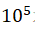m/s, and the velocity of a Yizi can exceed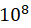m/s. (9) An additional interesting phenomenon is observed. When the mass M of a particle is considerably larger than the mass m of a different particle and the effect of the particle volumes is neglected, the momentum of the large-mass particle is equal to that of the small-mass particle, i.e.,Mv=mV ①.

Then, the velocity V of the small-mass particle is considerably higher than the velocity v of the large-mass particle, i.e., whenM≫m,v≪V ②.

From ① and ②,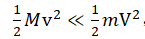, that is, the large-mass particle is in a very low temperature state, and the small-mass particle is in a very high temperature state. Consider that the small- and large-mass particles have equal kinetic energies, i.e.,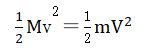③.

From ② and ③,Mv≫mV, and both particles are in a very high temperature state.

The abovementioned phenomenon shows that when two elementary particles with different masses are mixed together, the momenta of the two particles tend to equalize, and the temperature of the large-mass particle is lower than that of the small-mass particle. At this point, if these two elementary particles of different masses are completely separated, the large-mass particle can absorb the energy of the small-mass particle until the temperatures of the two particles equalize, such that the kinetic energies of the two particles tend to equalize.

Similarly, the temperature of a proton in a mixture of many protons and many Yizi particles is not the same as that when these protons and Yizi particles are completely separated. When a single proton suspended in a Yizi gas collides with the surrounding Yizi particles, the proton momentum tends to become equal to the average momentum of the Yizi particles, i.e.,Mz×VzMy×Vy ④.

The mass of the proton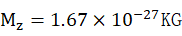, the mass of Yizi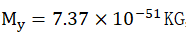, and the average velocity of Yizi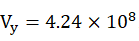m/s. The average velocity of the proton can be obtained from ④ as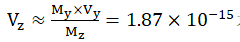m/s, and the corresponding proton temperature is close to absolute zero. If the mass densities of the protons, electrons, and Yizi particles are the same, the volume of a proton could be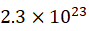times that of the Yizi. Therefore, the influence of the proton volume must be considered when many protons collect together and are completely separated from the Yizi gas that fills cosmic space. Each proton can absorb the kinetic energy of the Yizi particles in the Yizi gas, whereby the kinetic energy of each proton becomes equal to the sum of the kinetic energies of N Yizi particles, i.e.,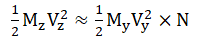⑤, where N is equal to the frequency of gamma rays produced by the self-collision of protons. If the frequency of gamma rays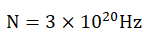, then the average velocity of the proton from ⑤ is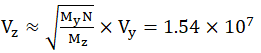m/s.

In this case, the temperature of the proton is equal to the central temperature of the sun, the central temperature of the earth, and the temperature in an atomic nucleus with a large atomic weight. The center of the sun and the center of the earth, like the nucleus of an atom, are made of protons. In fact, the nuclei of solid matter emit gamma rays at every moment, but most of the energy of the gamma rays is absorbed by the outer electrons of the nucleus, which release this energy in the form of thermal radiation. The use of X- and γ-ray meters to determine the air-kerma rate of the solid matter shows that all the solid matter around us always radiates X- and γ-rays at dose rates generally below0.25μSv/h. A Yizi transfers kinetic energy to protons via direct collision, which is then released as EM waves to maintain a dynamic balance. The cosmic space is not empty but full of Yizi gas. The energy of the Yizi gas per unit space volume is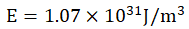, that is, the cosmic space is full of energy. We do not feel this energy because there is no energy difference between our bodies and cosmic space, where all the energy in the universe is kinetic energy. The Yizi gas contains an enormous quantity of energy and is at a super-high pressure. Fish in deep water do not feel hydrostatic pressure, and birds that land on high-voltage transmission lines do not feel electricity; similarly, we also do not feel the enormous energy and super-high pressure in space. (10) The high-pressure Yizi gas squeezes multiple protons into an atomic nucleus and constrains electrons to remain at the surface of this nucleus. The number of electrons outside the atomic nucleus is mainly related to the surface area of the nucleus, i.e., the larger the surface area of the nucleus is, the more electrons can be bound. Electrons and protons do not possess electricity. If this hypothesis is correct, there should be a wavelength associated with the fluctuation curve of an electron. Electrons could produce double-slit interference and single-slit diffraction just like visible light, but the fluctuations of the electrons should produce an electron wave with a wavelength much longer than the wavelength of the matter wave of electrons, for which experimental evidence is presented in the following sections.

1. Two experiments on the wave properties of electrons

1.1. Double-slit interference experiment on long-wavelength electrons

1.1.1. Equipment: (1) Vacuum tank: internal diameter, 180 cm; internal height, 180 cm; and material, steel plate. (2) Molecular pumps for generating a vacuum up to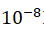Pa. (3) Negative direct current (DC) high-voltage power supply: high voltages up to -30,000 V and currents up to 1.4 mA. (4) Cathode emission source: a 3-mm-diameter stainless steel rod. (5) Two slits are made in a 20-cm-long, 15-cm-wide, and 0.5-mm-thick plastic plate. The width and length of the slits are 3 mm and 10 cm, respectively, and the distance between the two slits is 4 mm. The plastic plate is embedded in a 90-cm-wide, 120-cm-high, and 2-mm-thick organic glass plate. (6) An interferometry fringe display screen: one side is a plexiglass plate, and the other side is a steel ruler connected to the ground. Figure 2 shows the setup of the double-slit interference and single-slit diffraction experiments.Figure 2 Setup of the electron double-slit interference and single-slit diffraction experiments

1.1.2. Experimental principle: The electrons emitted by the cathode emission source can be regarded as wave sources with the same vibration direction, the same frequency, and a constant phase difference. The principle for obtaining fringes of coherent electrons and wavelength measurement is shown in Figure 3.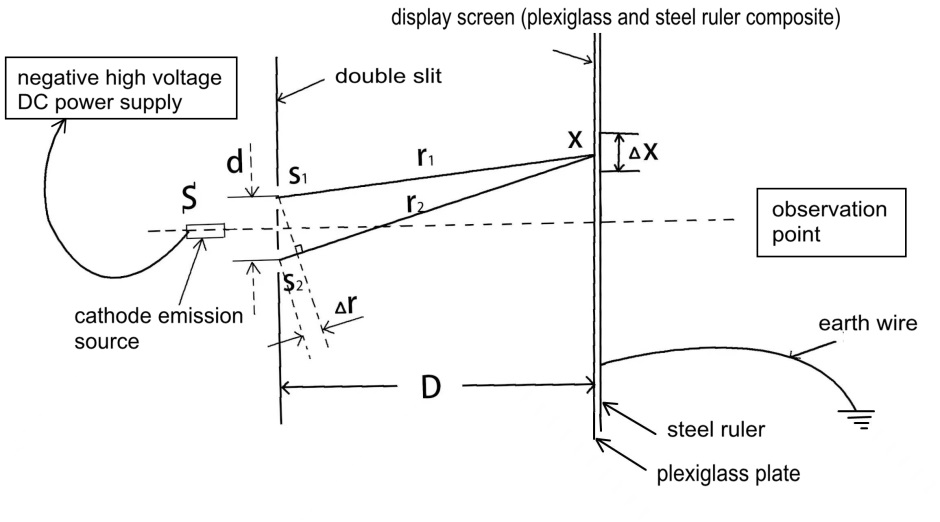Figure 3 Principle of double-slit electron interference

The cathode source S emits electrons with a wavelength of λ that pass through the double slits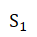and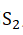with a spacing d. Some of these electrons hit the plexiglass plate of the display screen, and other electrons are absorbed by the steel ruler behind the organic glass, forming an interference pattern at the upper edge of the steel ruler. The distance between the interference fringes is denoted as ∆x, and the distance between the double slits and the display screen is denoted as D. Then, the wavelength of the electron wave is approximately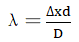.

1.1.3. Experimental procedure: (1) The cathode emission source, the plastic plate with double slits, and the display screen made of plexiglass plate and steel ruler are placed in sequence in a vacuum tank. (2) The steel ruler is connected to the ground, and the vacuum tank is closed. (3) The vacuum pump is turned on. When the absolute pressure in the vacuum tank reaches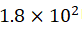Pa, the negative DC high-voltage power supply is turned on. When the voltage reaches -1710 V (U=1710 V) and the current reaches -1.23 mA, the cathode emission source starts to emit stable cathode rays. (4) The electrons emitted by the cathode source pass through the double slits. Some electrons hit the plexiglass plate of the screen, and some electrons bypass the organic glass and are absorbed by the steel ruler to create an interference pattern at the upper edge of the steel ruler, as shown in Figure 4.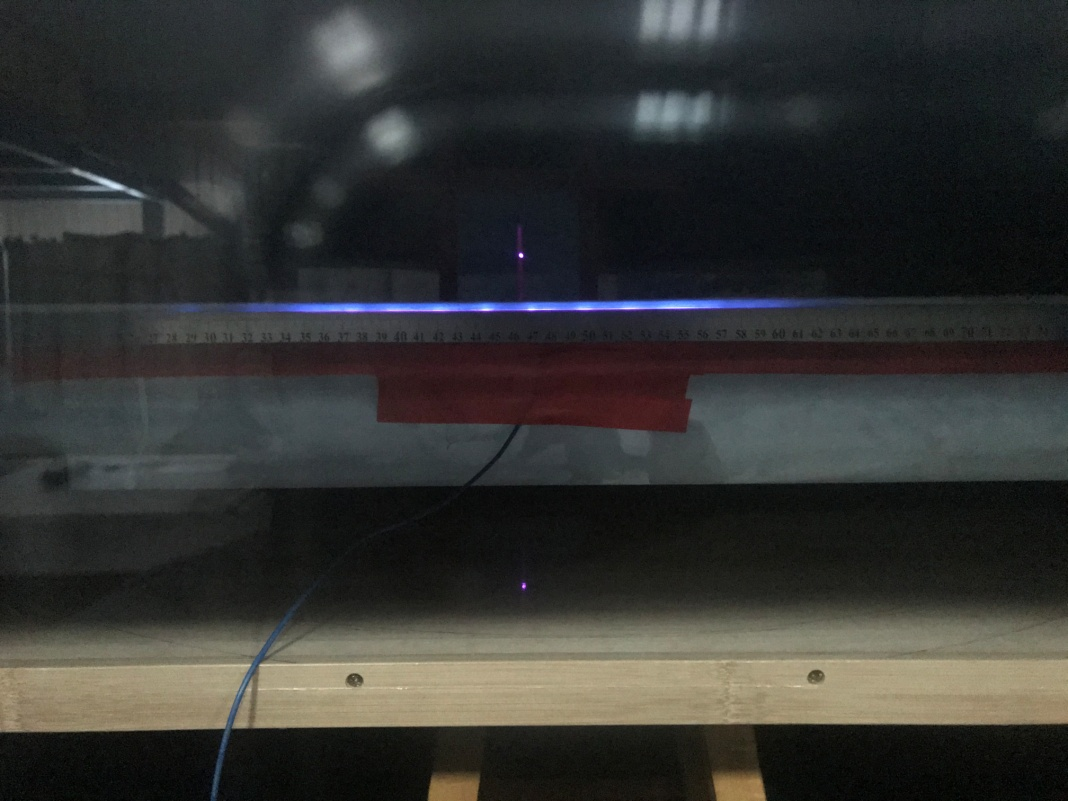Figure 4 Double-slit electron interference pattern

(5) Data and results: Given the simple test setup, only a qualitative analysis is conducted. The distance between the two slits is d = 7 mm, the distance between the two slits and the steel ruler is D = 400 mm, and the average distance between interference fringes is ∆x = 24.3 mm; hence,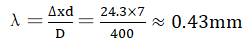.

The determined wavelength is not very accurate but nevertheless, quite different from the wavelength of matter waves. Changing the vacuum degree does not affect the fringes, indicating that the electrons have an inherent wave.

Neglecting the energy lost by electrons during wire transmission yields the kinetic energy of the electrons emitted from the cathode emission source as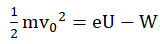, where m is the electron mass,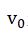is the linear velocity of the electrons after escaping from the cathode emission source, e is the electron charge, U is the voltage applied to the cathode emission source, and W is the work function required for electrons to escape from the cathode emission source. Neglecting W, which is usually small (e.g., typically, the metal work function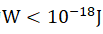), changes the formula presented above to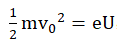, where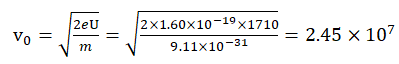m/s, the propagation speed of an electron wave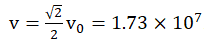m/s, and the frequency of an electron wave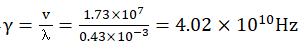.

1.2. Single-slit diffraction experiment of electrons with a long wavelength

1.2.1. Equipment: (1) Vacuum tank: internal diameter, 180 cm; internal height, 180 cm; and material, steel plate. (2) Molecular pumps for generating a vacuum up toPa. (3) Negative DC high-voltage power supply: high voltages up to -30,000 V and currents up to 1.4 mA. (4) Cathode emission source S: an Al nail with an 8-mm cap diameter. (5) A 5-mm-wide single slit is engraved on a plexiglass plate with a width of 90 cm, a height of 120 cm and a thickness of 2 mm. (6) A diffraction fringe screen: one side is a strip-shaped plexiglass plate, and the other side is a steel ruler connected to the ground.

1.2.2. Experimental principle: The electrons emitted by the cathode emission source can be regarded as wave sources with the same vibration direction, the same frequency, and a constant phase difference. Figure 5 shows the principle used to obtain the first-order dark fringe of the diffraction fringes and measure the wavelength.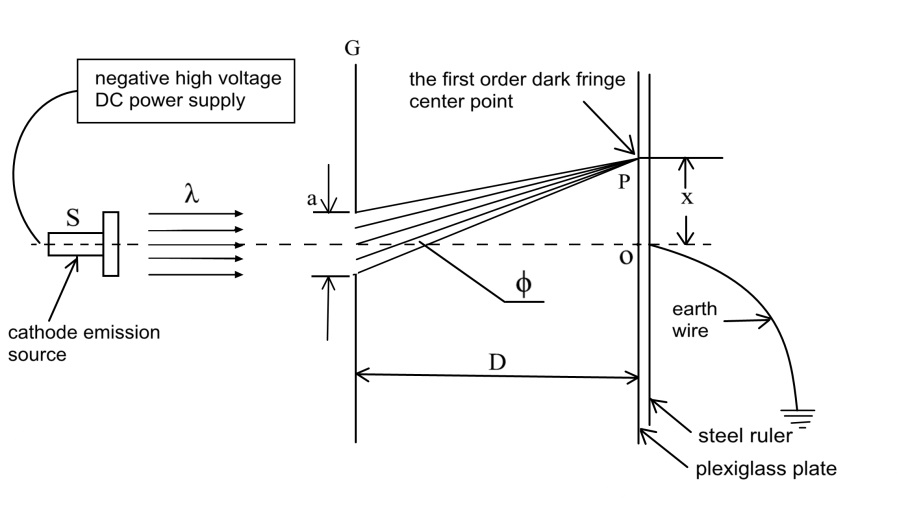Figure 5 Schematic of single slit diffraction

The cathode emission source S emits electrons with a wavelength λ that pass through the single slit G with spacing a. Some electrons hit the plexiglass plate of the display screen, and some electrons bypass the plexiglass plate and are absorbed by the steel ruler behind the plexiglass plate to create a diffraction pattern on the upper edge of the steel ruler. The distance from the center of the first dark fringe to the central axis is denoted as x, the diffraction angle is denoted asϕ, the distance from the single slit to the display screen is denoted as D, and the wavelength of the electron wave is approximately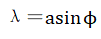,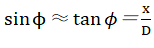,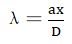.

1.2.3. Experimental procedure

(1) The cathode emission source, the plexiglass plate with a single slit, and the display screen made of the plexiglass plate and steel ruler are placed in sequence in a vacuum tank. The plexiglass plate with a single slit is approximately 10 cm from the cathode emission source and 360 mm from the display screen. (2) The steel ruler is connected to the ground, and the vacuum tank is closed. (3) The vacuum pump is turned on. When the absolute pressure in the vacuum tank reaches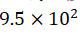Pa, the vacuum pump is turned off, and the negative DC high-voltage power supply is turned on. The voltage is slowly increased until the cathode emission source emits a stable cathode ray (the voltage reaches -2260 V (U = 2260 V) and the current reaches -0.7 mA). (4) The electrons pass through the single slit, some of which hit the organic glass on the display screen, and some which are absorbed by the steel ruler behind the organic glass. A diffraction pattern is formed at the upper edge of the steel ruler, as shown in Figures 6 and 7 (which show patterns obtained at night and during the day, respectively).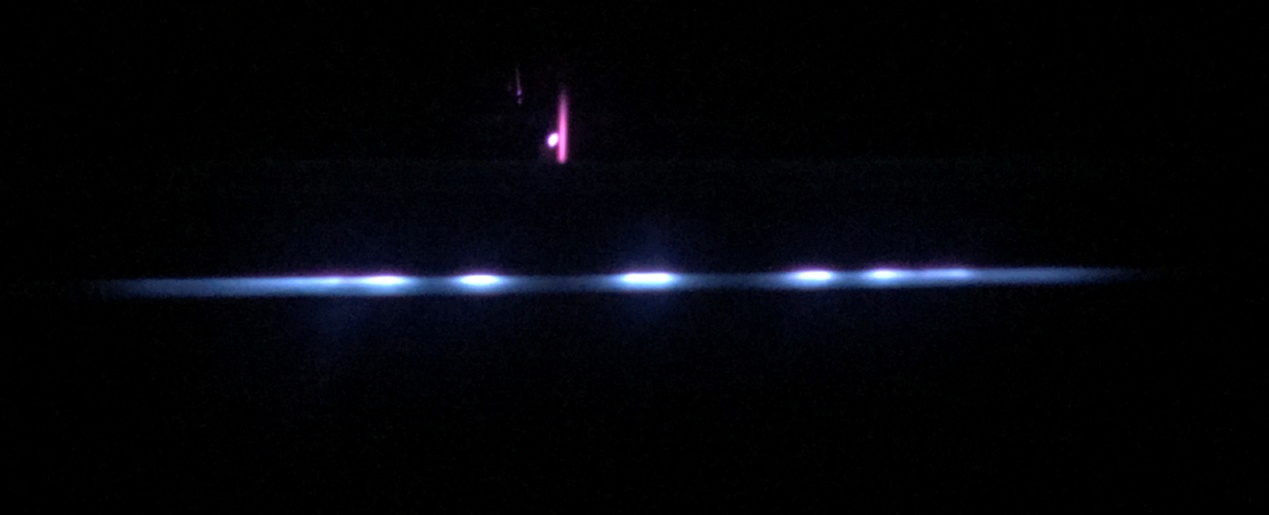Figure 6 Single-slit electron diffraction pattern (obtained at night)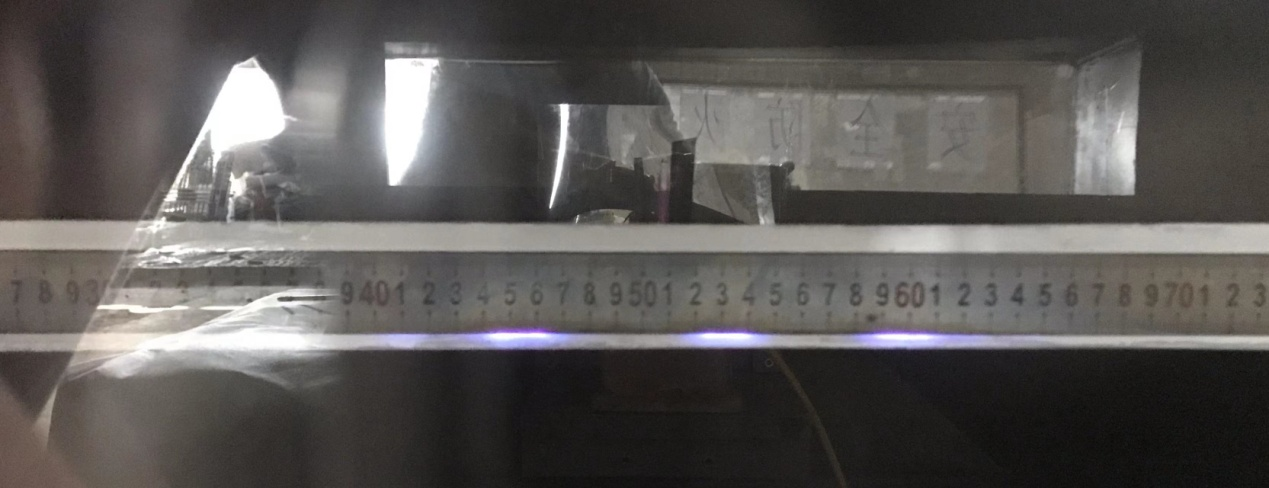Figure 7 Single-slit electron diffraction pattern (obtained during the day) at absolute pressure =Pa, U = -2260 V, and I = -0.7 mA

(5) Data and results: The width of the single slit is a = 5 mm, the distance from the single slit to the display screen is D = 360 mm, and the distance from the center of the first dark fringe to the central axis is x ≈ 35 mm; thus,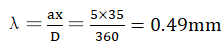. Given the simple test setup, the determined wavelength is not very accurate and is used only as a reference.

The linear velocity of the electrons that have escaped from the cathode emission source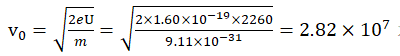m/s. The electron wave has a propagation speed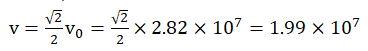m/s and a frequency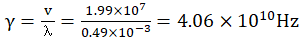.

(6) Increasing the vacuum in the vacuum tank causes the voltage to drop rapidly and the current to increase rapidly. As the allowable working current of the high-voltage DC power supply is ≤ 1.4 mA, the voltage of the high-voltage DC supply must be constantly reduced during the vacuum process to prevent the occurrence of an overcurrent. The vacuum pump is turned off when the absolute pressure in the vacuum tank reaches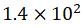Pa. At this moment, the voltage is -920 V (U = 920 V) and the current is -0.62 mA: the diffraction pattern of the electrons on the display screen is shown in Figure 8.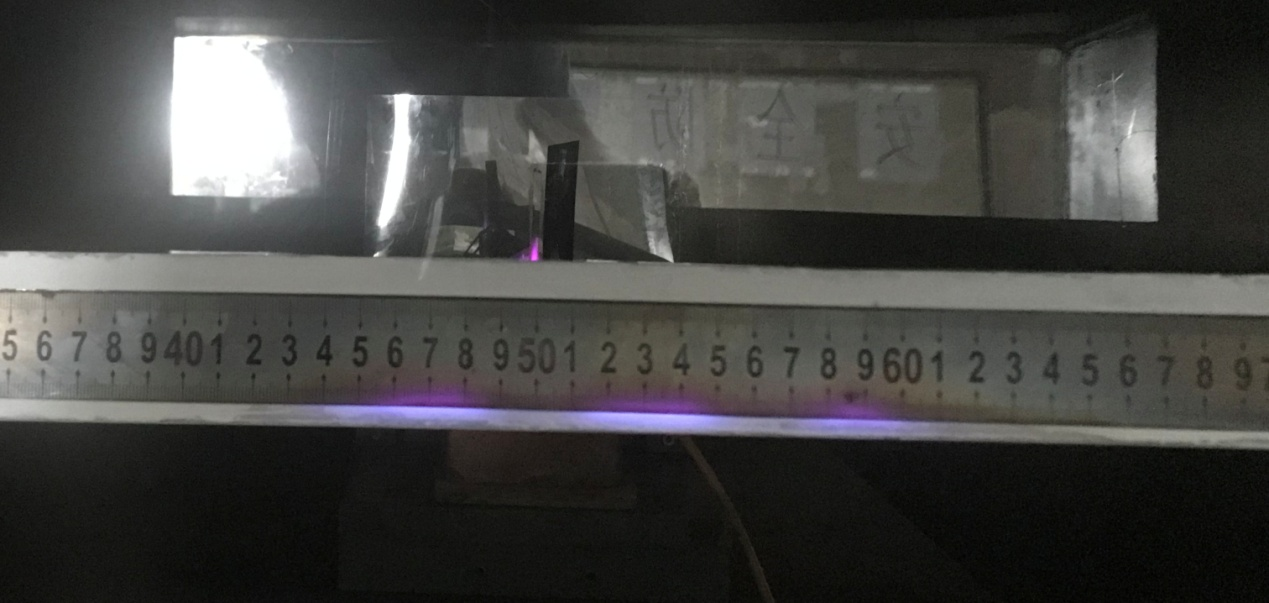(7) Data and results: The width of a single slit is a = 5 mm, the distance from the single slit to the display screen is D = 360 mm, and the distance from the center of the first dark fringe to the central axis is x ≈ 31 mm; thus,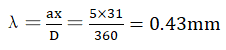. Given the simple test setup, the determined wavelength is not very accurate and is used only as a reference.

The linear velocity of the electrons that have escaped from the cathode emission source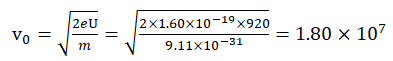m/s. The electron wave has a propagation speed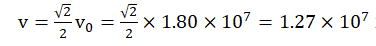m/s and a frequency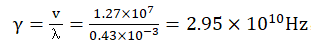. Both the wavelength and frequency of the electron wave decrease as the voltage decreases.

(8) Maintaining the absolute pressure atPa, the current is increased to 1.30 mA, resulting in a voltage of 1010 V. The electron single-slit diffraction pattern (obtained during the day) is shown in Figure 9.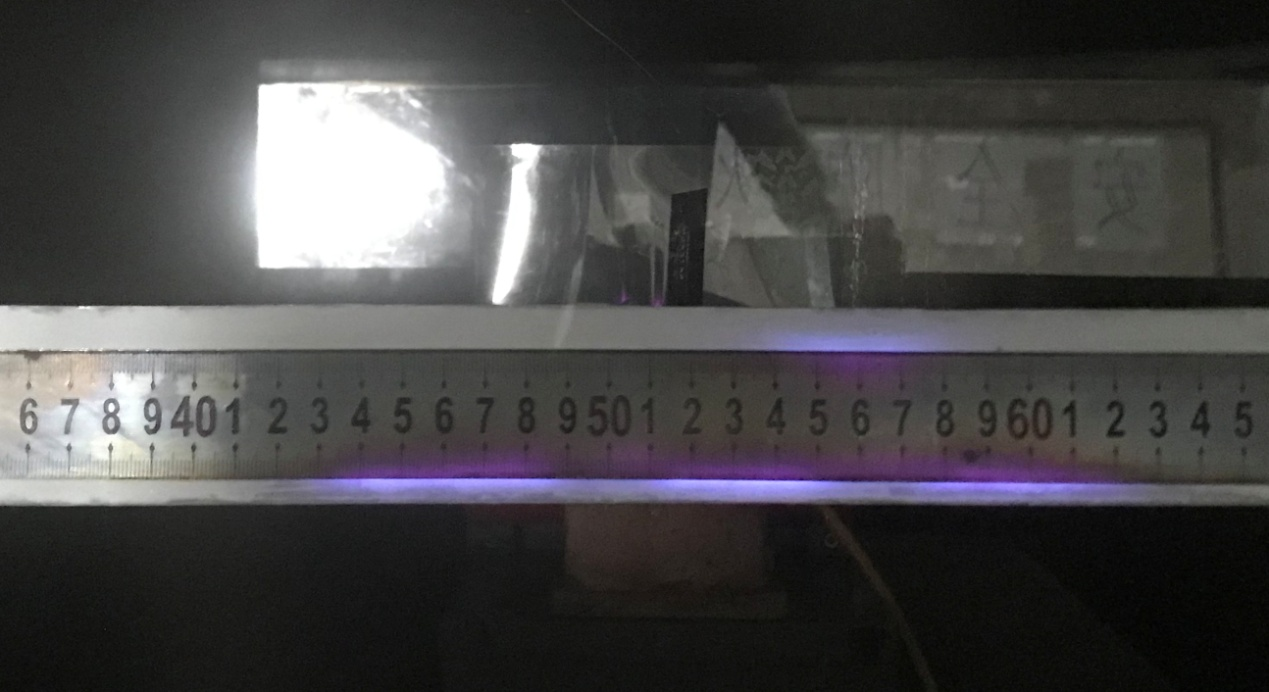Figure 9 Single-slit electron diffraction pattern (obtained during the day) at U = 1010 V and I = 1.30 mA

Comparing Figures 8 and 9 shows that a small increase in the voltage does not result in a significant change in the diffraction fringes even for a 2.1-fold increase in the current. If the frequency of an electron wave depends on the current, a 2.1-fold increase in the current should double the frequency of the electron wave. Thus, this experiment shows that the current has no effect on the single-slit diffraction of electrons.

(9)  Figures 7 and 8 show that a decrease in the pressure in the vacuum tank fromPa toPa does not result in a noticeable change in the diffraction fringes, indicating that the density of the residual gas does not affect the single-slit diffraction of electrons.

2. Experimental considerations

(1) The optimal slit width in both the double-slit interference and single-slit diffraction experiments ranges from 3 mm to 5 mm. (2) When the absolute pressure in the vacuum tank is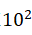-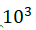Pa, clear fringes are observed in the double-slit interference and single-slit diffraction experiments. The absolute pressure should not be too small. At absolute pressures below 16 Pa, the electrons emitted by the cathode emission source can diffuse like a gas, and a cathode ray cannot be produced. (3) In both the double-slit interference and single-slit diffraction experiments, both double and single slits should be embedded in a large insulating plate to prevent the cathode rays from bypassing the double or single slit to reach the display screen.

3. Conclusions

(1) The physical meaning of the matter wave is as follows. Simply speaking, when an object moves in a gas, a matter wave is generated as a fluctuation due to the continuous resistance of the particles constituting the gas, that is, a matter wave is a probability wave. When an object moves in a Yizi gas, EM waves are generated, and the object itself moves forward in a wavelike manner. The kinetic energy of an object represents the ability of electrons to occupy a space (volume). A fixed quantity of energy can be contained in a unit volume of space. The higher the kinetic energy of an object, the more volume the object occupies in space, the higher the number of collisions with Yizi particles per unit time is, the shorter the wavelength of the matter wave is, and the higher the frequency of the matter wave is. Figure 10 shows the idealized trajectory of an electron. However, a probability wave is generated by the collision between the electrons moving in a Yizi gas and the Yizi particles. Transforming the equation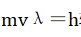to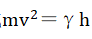and letting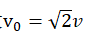yields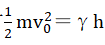.

In the formulas given above, m is the mass of an electron; v is the wave velocity of the electron matter wave; λ is the wavelength of the matter wave; h is Planck’s constant, i.e., the average kinetic energy of the Yizi particles; γ is the frequency of the matter wave, which represents the number of Yizi particles in the collision; andis the average speed of the electrons. The velocity v in the mass wave equationmvλ=h is not equal to the average velocityof the moving particle: instead,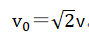. The Yizi gas interferes with the motion of electrons, resulting in the generation of matter waves, the uncertainty principle , and the difference between the moving mass and static mass.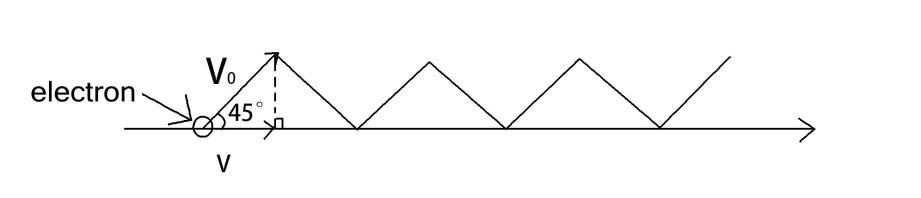Figure 10 Idealized trajectory of an electron

(2) An electron wave is generated by the periodic curvilinear motion of the eccentric rotation of an electron. This eccentric rotation prevents electrons from moving uniformly and linearly in the absence of an external force (e.g., such as that exerted by macroscopic objects) and results in the wave-particle duality of electrons.

The most significant feature of electron waves is that the wavelength and frequency of the electron increase simultaneously with the velocity.

(3) A characteristic common to both the electron matter wave and electron wave is that the wavelength and frequency are independent of the current.

(4) The wavelength measured in the double-slit interference and the single-slit diffraction experiments in this study is 0.43 mm and 0.49 mm, respectively. By comparison, in the 1925 Davisson-Germer electron diffraction experiment, the wavelength of the matter wave of an incident electron was measured asλ=0.167 nm. Taking double-slit interference as an example, the wavelength of the electron wave is approximately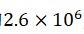times the wavelength of the matter wave, that is, the wavelengths of the electron wave and matter wave are not on the same order of magnitude.

The matter wave and electron wave have completely different properties. The matter wave is the fluctuation generated by the collision of a moving object with Yizi gas particles and incorporates everything moving in the Yizi gas, including the object. The electron wave is unique to elementary particles and results from the eccentric rotation of electrons. Macroscopic objects do not undergo eccentric rotation and therefore cannot form this unique wave.

References

 Y. S. Chen, Z. Z. Li, College Physics (Tianjin University, Tianjin, 1999).

 H.W. Li, Physics Essays 34. 2, 236 (2021).

 H. W. Li, Physics Essays 34. 2, 236 (2021).

 Y. S. Chen, Z. Z. Li, College Physics (Tianjin University, Tianjin, 1999).

 Y. S. Chen, Z. Z. Li, College Physics (Tianjin University, Tianjin, 1999).

 Y. S. Chen, Z. Z. Li, College Physics (Tianjin University, Tianjin, 1999).

 上一页      回目录      下一页
 ------------------------------------------------------------------------------------------------------------------------------------------------
 Copyright (C) 2004 rights reserved.李华旺 版权所有 电话:086-0411-82641525 E-mail:lhw@dlhuaxin.com 易子论网（李华旺） 地址:中国大连中山区明泽街16号 丽苑大厦5楼H座 邮编:116001 Author: li Huawang Address: 5H, Liyuan Mansion, No.16, Mingze Street, Zhongshan District, Dalian City Postcode: 116001 E-mail:lhw@dlhuaxin.com 辽ICP备12005732号